# Difference between revisions of "Limit theorems"

in probability theory

2010 Mathematics Subject Classification: Primary: 60Fxx [MSN][ZBL]

A general name for a number of theorems in probability theory that give conditions for the appearance of some regularity as the result of the action of a large number of random sources. The first limit theorems, established by J. Bernoulli (1713) and P. Laplace (1812), are related to the distribution of the deviation of the frequencyof appearance of some eventinindependent trials from its probability,(exact statements can be found in the articles Bernoulli theorem; Laplace theorem). S. Poisson (1837) generalized these theorems to the case when the probability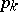of appearance ofin the-th trial depends on, by writing down the limiting behaviour, as, of the distribution of the deviation of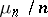from the arithmetic mean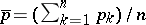of the probabilities,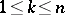(cf. Poisson theorem). Ifdenotes the random variable that takes the value 1 ifappears in the-th trial and the value 0 when the opposite event appears, then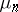can be expressed as the sum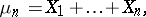which makes it possible to regard the theorems mentioned above as particular cases of two more general statements related to sums of independent random variables — the law of large numbers and the central limit theorem (these are given in their classical forms below).

## The law of large numbers.

Let(1)

be a sequence of independent random variables, let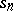be the sum of the firstelements of this sequence,(2)

let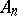andbe, respectively, the mathematical expectation,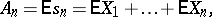and variance (cf. Dispersion),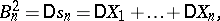of the sum. One says that the sequence (1) is subject to the law of large numbers if, for any, the probability of the inequalitytends to zero as.

Very general conditions for the law of large numbers to be applicable were found first by P.L. Chebyshev (1867) and were later generalized by A.A. Markov (1906). The problem of necessary and sufficient conditions for the law of large numbers to be applicable was exhaustively treated by A.N. Kolmogorov (1928). If all random variables have the same distribution function, then these conditions reduce to one: the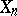must have finite mathematical expectation (this was shown by A.Ya. Khinchin in 1929).

## The central limit theorem.

One says that the central limit theorem holds for a sequence (1) if for arbitrary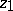and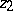the probability of the inequality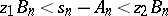has as limit, as, the quantity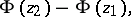where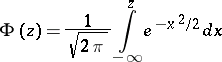(cf. Normal distribution). Rather general sufficient conditions for the central limit theorem to hold were indicated by Chebyshev (1887); however, his proofs contained gaps, which were filled in somewhat later by Markov (1898). A solution of the problem which is nearly final was obtained by A.M. Lyapunov (1901). The exact formulation of Lyapunov's theorem is: Suppose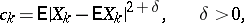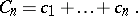If the ratio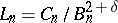tends to zero as, then the central limit theorem holds for (1). The final solution to the problem of conditions of applicability of the central limit theorem was obtained, in general outline, by S.N. Bernstein [S.N. Bernshtein] (1926) and was completed by W. Feller (1935). Under the conditions of the central limit theorem the relative accuracy of approximation of the probability of an inequality of the form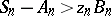, wheregrows unboundedly astends to infinity, by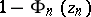can be very low. Correction factors necessary in order to increase the accuracy are indicated in limit theorems for probabilities of large deviations (cf. Probability of large deviations; Cramér theorem). This question was studied, following H. Cramér and Feller, by Yu.V. Linnik and others. Typical results related to this branch of the subject are most conveniently explained using the example of the sums (2) of independent identically-distributed random variables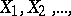with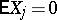and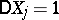. In this case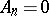,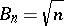.

Consider, e.g., the probability of the inequalitywhich equals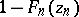, where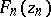is the distribution function of the variable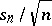, and for fixed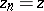as,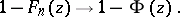(3)

If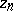depends onand is moreover such that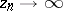as, then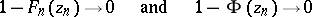and formula (3) is useless. It is necessary to obtain bounds on the relative accuracy of approximation, i.e. for the ratio ofto. In particular, there naturally arises the question of conditions under which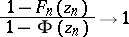(4)

as.

Relation (4) holds forof arbitrary growth only if the summands have a normal distribution (this conclusion is valid as soon asis of order greater than). If the summands are not normal, then relation (4) can hold in certain zones, whose orders do not exceed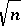. "Smaller" zones (of logarithmic order) are obtained under the condition that a certain number of moments is finite. Moreover, under definite "regularity" conditions on the densities of the summands the "normality" asymptotics imply power asymptotics. E.g., if the density of the summands is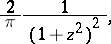then, uniformly in, as,Taking into account that as,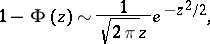it is easy to convince oneself that (4) holds. The extension to zones of power order (of the form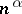,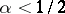), requires that the condition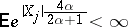(5)

should hold, as well as that a certain number (depending on) of moments of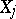coincide with corresponding moments of the normal distribution. If the latter condition (on the coincidence of moments) is not satisfied, then the ratio at the left-hand side of (4) can be described by a Cramér series (under the so-called Cramér condition, cf. Cramér theorem) or initial partial sums of it (under a condition of the type (5)).

Estimates of probabilities of large deviations are used in mathematical statistics, statistical physics, etc.

The following may be distinguished among the other directions of research in the domain of limit theorems.

1) Research, initiated by Markov and continued by Bernstein and others, on conditions under which the law of large numbers and the central limit theorem hold for sums of dependent random variables.

2) Even in the case of sequences of identically-distributed random variables one can exhibit simple examples when "normalized" (i.e. subjected to a certain linear transformation) sums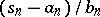,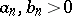constants, have a limit distribution different from a normal one (non-degenerate distributions, i.e. distributions not concentrated at a single point, are meant) (cf. Stable distribution). In the work of Khinchin, B.V. Gnedenko, P. Lévy, W. Doeblin, and others, both the class of possible distributions for sums of independent random variables and conditions of convergence of the distributions of sums to some limit distribution (in triangular arrays (cf. Triangular array) of random variables one imposes here the condition of asymptotic negligibility of the summands) have been completely studied. (Cf. Infinitely-divisible distribution; Stochastic process with independent increments.)

3) Local limit theorems have been given considerable attention. E.g., suppose that the random variablestake only integer values. Then the sumsalso take integer values only, and it is natural to pose the question of the limiting behaviour of the probabilitiesthat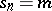, whereis an integer. The simplest example of a local limit theorem is the local Laplace theorem. Another type of local limit theorem describes the limiting distribution of the densities of the distributions of sums.

4) Limit theorems in their classical formulation describe the behaviour of individual sumsasgrows. Sufficiently general limit theorems for probabilities of events depending on several sums at once were first obtained by Kolmogorov (1931). His results imply, e.g., that under fairly general conditions the probability of the inequality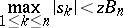has as limit the quantity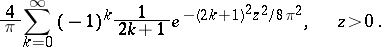A most general means for proving analogous limit theorems is by limit transition from discrete to continuous processes.

5) The limit theorems given above are related to sums of random variables. An example of a limit theorem of different kind is given by limit theorems for order statistics. These theorems have been studied in detail by Gnedenko, N.V. Smirnov and others.

6) Finally, theorems establishing properties of sequences of random variables occurring with probability one are called strong limit theorems. (Cf. Strong law of large numbers; Law of the iterated logarithm.)

For methods of proof of limit theorems see Characteristic function; Distributions, convergence of.